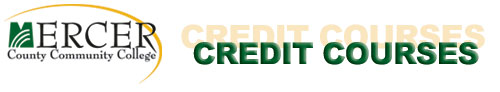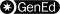(MAT) Mathematics

[program website]

Note: Students should check mathematics course recommendations with the college or university to which they intend to transfer. The receiving institution always makes the final decision concerning transferability of credits. Information about mathematics course equivalencies among New Jersey institutions is available at www.njtransfer.org.

For initial selection of a mathematics course, check with the Math Department. Students who have completed math courses at another college must present transcripts and course outlines or syllabi. Consult mathematics faculty for advice.

Applicability of credits for courses below the 100 level toward degree requirements is limited. Consult an academic advisor. All prerequisite courses must be passed with a minimum C grade before enrolling in any subsequent mathematics course.MAT 033 - Pre-Algebra (4 credits)
Developmental mathematics course designed for students needing a review of basic arithmetic, including an introduction to algebra. Topics include operations with whole numbers, decimals, fractions, percents, ratio and proportion, signed numbers, and an introduction to algebraic equations. [Foundation course does not fulfill mathematics elective requirement.] 4 lecture hours
course outlineMAT 037 - Beginning Algebra (4 credits)
Prerequisite: MAT 033 with a minimum C grade or appropriate placement test score
Foundation mathematics course designed for students with experience in algebra but who need to strengthen their mastery of the fundamentals. Topics include exponents, polynomials, factoring, graphing first-degree equations, quadratic equations, rational expressions, and radical expressions. [Foundation course does not fulfill mathematics elective requirement.] 4 lecture hours
course outlineMAT 041 - Foundation Math I (3 credits)
Developmental mathematics course designed for students needing a review of basic arithmetic, including an introduction to algebra. Topics include whole numbers, fractions, decimals, percentages, and integer operations. Students work through the material in self-paced mastery-based modules in a lab setting. [Foundation course does not fulfill mathematics elective requirement.] 6 laboratory hours
course outlineMAT 042 - Foundation Math II (3 credits)
Prerequisite: MAT 041
Developmental mathematics course designed for students needing an introduction to algebra. Topics include inequalities, linear models and graphing, exponents, and polynomials. Students work through the material in mastery-based modules in a lab setting. Those who complete this course may register for MAT 115, MAT 120, MAT 125, or MAT 140. [Foundation course does not fulfill mathematics elective requirement.] 6 laboratory hours
course outlineMAT 043 - Foundation Math III (1 credit)
Prerequisite: MAT 042
Developmental mathematics course designed for students needing additional topics in algebra. Students work through the material - operations on rational and radical expressions, systems of equations, and linear models - in mastery-based modules in a lab setting. Those who complete this course may register for MAT 135 or MAT 140. [Foundation course does not fulfill mathematics elective requirement.] 2 laboratory hours
course outlineMathematics MAT 115 - Algebra and Trigonometry I (3 credits) Prerequisite: MAT 037 (or MAT 037A and 037B) or MAT 042 or appropriate placement test score Primarily for students majoring in engineering technology related programs. Algebraic topics discussed include systems of linear equations, determinants, factoring, trigonometric functions and their graphs, radian measure, solutions of triangles, and application problems. 3 lecture hours course outlineMathematics MAT 116 - Algebra and Trigonometry II (3 credits) Prerequisite: MAT 115 with a minimum C grade or permission of the Mathematics Department Continuation of MAT 115. Topics include complex numbers, logarithmic and exponential functions, solving systems of nonlinear equations, trigonometric identities and equations, inverse trigonometric functions, and analytic geometry. 3 lecture hours course outlineMathematics MAT 120 - Mathematics for Liberal Arts (3 credits) Prerequisite: MAT 037 (or MAT 037A and 037B) or MAT 042 or appropriate placement test score Primarily for students in non-scientific/non-technical majors, emphasizes mathematical systems, reasoning, and mathematical structures. Includes sets, symbolic logic, numeration systems, number systems in other bases, growth models, and geometric structures. 3 lecture hours course outlineMathematics MAT 125 - Elementary Statistics I (3 credits) Prerequisite: MAT 037 (or MAT 037A and 037B) or MAT 042 with a minimum C grade or appropriate placement test score A basic introduction to statistical concepts and methods. Topics include descriptive statistics, basic probability concepts, discrete and normal probability distributions, hypothesis testing and confidence intervals with one sample mean and one sample proportion, as well as regression and correlation. Studies include the use of statistical software. 3 lecture hours course outlineMathematics MAT 126 - Elementary Statistics II (3 credits) Prerequisite: MAT 125 Continuation of MAT 125. Topics include random sampling, experimental and observational studies, fundamentals of probability, confidence intervals and hypothesis testing on two populations and two proportions, F and Chi-Square distributions, analysis of variance, and basic nonparametric tests. Studies include the use of statistical software. 3 lecture hours course outlineMathematics MAT 135 - Intermediate Algebra with Applications (4 credits) Prerequisite: MAT 037 (or MAT 037A and 037B) or MAT 043 with a minimum C grade or appropriate placement test score Topics include function notation; linear, quadratic, and absolute value functions and equations; rational expressions and equations; rational exponents and equations; radicals and radical equations; graphing of linear, quadratic, and polynomial functions; and inequalities. [grade of B- or better is strongly recommended to proceed to MAT 146] 4 lecture hours course outlineMathematics MAT 140 - Applied College Algebra (4 credits) Prerequisite: MAT 037 (or MAT 037A and 037B) or MAT 042 with a minimum C grade or appropriate placement test score Designed for students majoring in disciplines involving less intensive math, for which a more conceptual understanding of college algebra is appropriate. Employing extensive examples from a variety of fields, topics include the study of linear, exponential, logarithmic, polynomial and rational functions. Not intended as preparation for Pre-Calculus or Calculus. 4 lecture hours. course outlineMathematics MAT 146 - Pre-Calculus (4 credits) Prerequisite: MAT 135 with a minimum C grade or appropriate College Level Math placement test score In-depth study of polynomial, rational, exponential, logarithmic, trigonometric and inverse trigonometric functions, equations, and identities; systems of equations including matrices; extensive use of graphing calculators. [grade of B- or better is strongly recommended to proceed to MAT 151] 4 lecture hours course outlineMathematics MAT 149 - Calculus (4 credits) Prerequisite: MAT 146 with a minimum C grade or appropriate College Level Math placement test score Application-based topics include the fundamental techniques of differentiation and integration of algebraic, trigonometric, exponential and logarithmic functions. Study focuses on optimization, maxima-minima and marginal analysis for differentiation and includes substitution method among other specific integration techniques. Not intended as a prerequisite for MAT 152. 4 lecture hours course outlineMathematics MAT 151 - Calculus I for the Mathematical and Physical Sciences (4 credits) Prerequisite: MAT 146 with a minimum C grade or appropriate College Level Math placement test score First course in the standard integrated calculus sequence. Topics include differentiation of algebraic, exponential, logarithmic, trigonometric, hyperbolic, and inverse trigonometric functions. Applications include curve sketching, related rates, maxima, minima, and approximations as well as integration and applications of the definite integral. 4 lecture hours course outlineMathematics MAT 152 - Calculus II for the Mathematical and Physical Sciences (4 credits) Prerequisite: MAT 151 with a minimum C grade and consultation with mathematics faculty member Continuation of MAT 151. Topics include techniques of integration, areas, volumes, arc length, surface area, improper integrals, Simpson's Rule, infinite sequences, MacLaurin and Taylor series, differentiation of polar and parametric equations, conic sections in rectangular and polar form, and rotation of axes. 4 lecture hours course outlineMathematics MAT 200 - Statistics for Social and Health Sciences I (3 credits) Prerequisite: MAT 135 with a minimum C grade or appropriate College Level Math placement test score or permission of department An applied statistics course for the social sciences, nursing, etc. Topics include data production and access, one-variable data analysis, correlation and regression, normal and binomial distributions, sampling distributions, estimation and tests of hypotheses for a single sample. MINITAB statistical software is used to calculate statistics and generate graphs. 3 lecture hours course outlineMathematics MAT 201 - Probability and Statistics for Science and Engineering (4 credits) Prerequisite: MAT 151 or MAT 149 with a minimum C grade or permission of department Calculus-based course designed for engineers, computer scientists and science majors. Topics include one variable data analysis, sample regression analysis, probability, discrete and continuous distributions, random samples, confidence intervals and hypothesis testing, contingency tables, as well as one factor and factorial experimental design. 4 lecture hours course outlineMathematics MAT 205 - Statistics for Social and Health Sciences II (3 credits) Prerequisites: MAT 200 with a minimum C grade or permission of department Second-semester course in an academic year sequence for social sciences, humanities, nursing and related fields. Employs statistical software for studies of probability, inference for two parameters, inference for regression and correlation, analysis of variance, analysis of categorical data, and nonparametric statistics. [Spring offering] 3 lecture hours course outlineMathematics MAT 208 - Linear Algebra (4 credits) Prerequisite: MAT 151 with a minimum C grade and consultation with mathematics faculty member An introduction to linear algebra topics including linear equations and matrices, determinants, independence and basis, vector spaces and subspaces, the four fundamental subspaces, orthogonality, linear transformations and eigenvalues and eigenvectors. Applications of linear algebra are included. 4 lecture hours course outlineMathematics MAT 251 - Calculus III (4 credits) Prerequisite: MAT 152 with a minimum C grade and consultation with mathematics faculty member Continuation of MAT 152. Includes parametric equations, vectors, solid analytic geometry, partial derivatives, multiple integrals, and topics in vector calculus including Green's theorem and Stoke's theorem. 4 lecture hours course outlineMathematics MAT 252 - Differential Equations (4 credits) Prerequisite: MAT 152 with a minimum C grade and consultation with mathematics faculty member Topics include solutions of ordinary differential equations, solving linear differential equations of higher order using differential operators, methods of undetermined coefficients and variation of parameters. Strong emphasis on solving differential equations using the Laplace transform, Cauchy-Euler equation, infinite series, and matrix methods. Applications to geometry and physical science are discussed. 4 lecture hours course outline